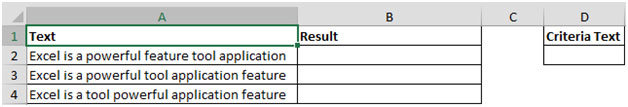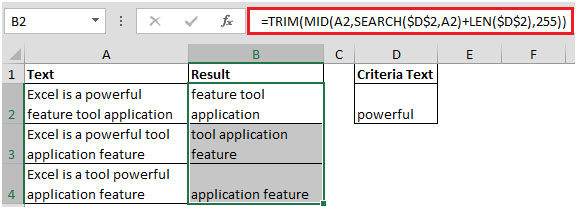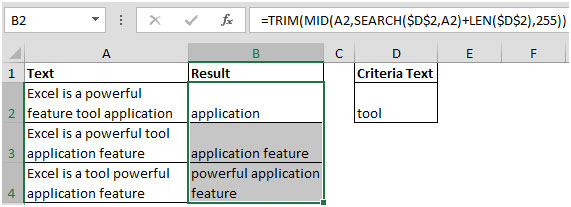# How To Extract All Text Strings After A Specific Text String In Microsoft Excel

In this article, you will learn how to extract all text strings after a specific text.

If there is a requirement to retrieve the data from a column after a specific text, we can use a combination of TRIM, MID, SEARCH, LEN functions to get the output.

Let us understand with an example:

• We have text in column A, and in column B, we want to get the result & cell D2 contains the criteria or lookup text. Refer below snapshot• Let us enter word “powerful” in Criteria Text cell D2
• The formula in cell B2 would be =TRIM(MID(A2,SEARCH(\$D\$2,A2)+LEN(\$D\$2),255))• If we change the Criteria Text say “tool” then, following will be the result (as shown in below screenshot):In this way, you can get the text strings after a specific text string.

1.how can i extract word after "powerfull" till "feature"

2.How would I extract 8 characters after the Criteria Text?

•Hey Neil,

Its an interesting question, Let's assume,word be in A3 cell , specific text be in D2 cell. You need extract n characters after the Criteria Text. Use this formula =TRIM(MID(A3,SEARCH(D2,A3)+(n+LEN(D2)),255)). In your case Just replace the n with 8.
=TRIM(MID(A3,SEARCH(D2,A3)+(n+LEN(D2)),255))

3.Hi, thanks for the tutorial. How would you extract text BEFORE a specific string?
Thanks

•Use this formula
=LEFT(text,FIND("specific character")-1)
•4.